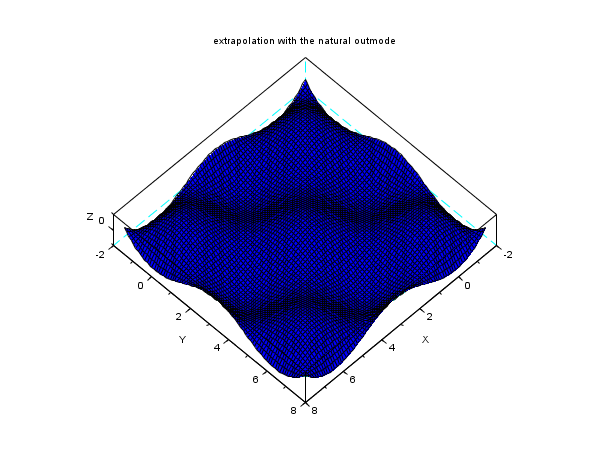Scilab Home page | Wiki | Bug tracker | Forge | Mailing list archives | ATOMS | File exchange
Change language to: English - Français - Português - 日本語 -

See the recommended documentation of this function

Справка Scilab >> Interpolation > interp2d

# interp2d

bicubic spline (2d) evaluation function

### Syntax

`[zp[, dzpdx, dzpdy[, d2zpdxx, d2zpdxy, d2zpdyy]]]=interp2d(xp, yp, x, y, C [,out_mode])`

### Arguments

xp

a mx-by-my matrix of doubles, the `x` coordinates of the points where the spline is to be evaluated.

yp

a mx-by-my matrix of doubles, the `y` coordinates of the points where the spline is to be evaluated.

x

a 1-by-nx matrix of doubles, the `x` coordinate of the interpolation points. We must have x(i)<x(i+1), for i=1,2,...,nx-1.

y

a 1-by-ny matrix of doubles, the `y` coordinate of the interpolation points. We must have y(i)<y(i+1), for i=1,2,...,ny-1.

C

The coefficients of the bicubic spline. The input argument of the interp2d function is the output argument of the splin2d function.

out_mode

a 1-by-1 matrix of strings, the evaluation of s outside the range [x(1),x(nx)] by [y(1),y(ny)].

zp

a mx-by-my matrix of doubles, the evaluation of the `z` coordinate of the spline, i.e. zp(i,j)=s(xp(i,j),yp(i,j)), for i=1,2,...,mx and j = 1,2,...,my.

dzpdx

a mx-by-my matrix of doubles, the first derivative of the spline with respect to `x`.

dzpdy

a mx-by-my matrix of doubles, the first derivative of the spline with respect to `y`.

d2zpdxx

a mx-by-my matrix of doubles, the second derivative of the spline with respect to `x`.

d2zpdxy

a mx-by-my matrix of doubles, the second derivative of the spline with respect to `x` and `y`.

d2zpdyy

a mx-by-my matrix of doubles, the second derivative of the spline with respect to `y`.

### Description

Given three vectors `(x,y,C)` defining a bicubic spline or sub-spline function (see splin2d) this function evaluates s (and ds/dx, ds/dy, d2s/dxx, d2s/dxy, d2s/dyy if needed) at (xp(i),yp(i)) :The `out_mode` parameter defines the evaluation rule for extrapolation, i.e. for (xp(i),yp(i)) not in [x(1),x(nx)]x[y(1),y(ny)] :

"by_zero"

an extrapolation by zero is done

"by_nan"

extrapolation by Nan

"C0"

the extrapolation is defined as follows :

```s(x,y) = s(proj(x,y)) where proj(x,y) is nearest point
of [x(1),x(nx)]x[y(1),y(ny)] from (x,y)```
"natural"

the extrapolation is done by using the nearest bicubic-patch from (x,y).

"periodic"

`s` is extended by periodicity.

### Examples

```n = 7;  // a n x n interpolation grid
x = linspace(0,2*%pi,n); y = x;
z = cos(x')*cos(y);
C = splin2d(x, y, z, "periodic");

// now evaluate on a bigger domain than [0,2pi]x [0,2pi]
m = 80; // discretization parameter of the evaluation grid
xx = linspace(-0.5*%pi,2.5*%pi,m); yy = xx;
[XX,YY] = ndgrid(xx,yy);
zz1 = interp2d(XX,YY, x, y, C, "C0");
plot3d(xx, yy, zz1, flag=[2 6 4])
xtitle("extrapolation with the C0 outmode")``````n = 7;  // a n x n interpolation grid
x = linspace(0,2*%pi,n); y = x;
z = cos(x')*cos(y);
C = splin2d(x, y, z, "periodic");

// now evaluate on a bigger domain than [0,2pi]x [0,2pi]
m = 80; // discretization parameter of the evaluation grid
xx = linspace(-0.5*%pi,2.5*%pi,m); yy = xx;
[XX,YY] = ndgrid(xx,yy);
zz2 = interp2d(XX,YY, x, y, C, "by_zero");
plot3d(xx, yy, zz2, flag=[2 6 4])
xtitle("extrapolation with the by_zero outmode")``````n = 7;  // a n x n interpolation grid
x = linspace(0,2*%pi,n); y = x;
z = cos(x')*cos(y);
C = splin2d(x, y, z, "periodic");

// now evaluate on a bigger domain than [0,2pi]x [0,2pi]
m = 80; // discretization parameter of the evaluation grid
xx = linspace(-0.5*%pi,2.5*%pi,m); yy = xx;
[XX,YY] = ndgrid(xx,yy);
zz3 = interp2d(XX,YY, x, y, C, "periodic");
plot3d(xx, yy, zz3, flag=[2 6 4])
xtitle("extrapolation with the periodic outmode")``````n = 7;  // a n x n interpolation grid
x = linspace(0,2*%pi,n); y = x;
z = cos(x')*cos(y);
C = splin2d(x, y, z, "periodic");

// now evaluate on a bigger domain than [0,2pi]x [0,2pi]
m = 80; // discretization parameter of the evaluation grid
xx = linspace(-0.5*%pi,2.5*%pi,m); yy = xx;
[XX,YY] = ndgrid(xx,yy);
zz4 = interp2d(XX,YY, x, y, C, "natural");
plot3d(xx, yy, zz4, flag=[2 6 4])
xtitle("extrapolation with the natural outmode")```• splin2d — bicubic spline gridded 2d interpolation

### History

 Version Description 5.4.0 Previously, imaginary part of input arguments were implicitly ignored.